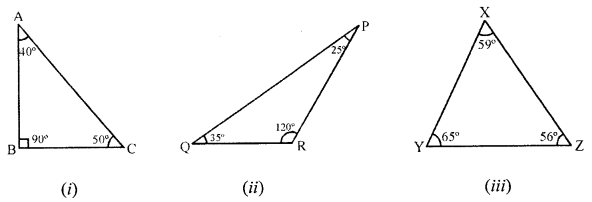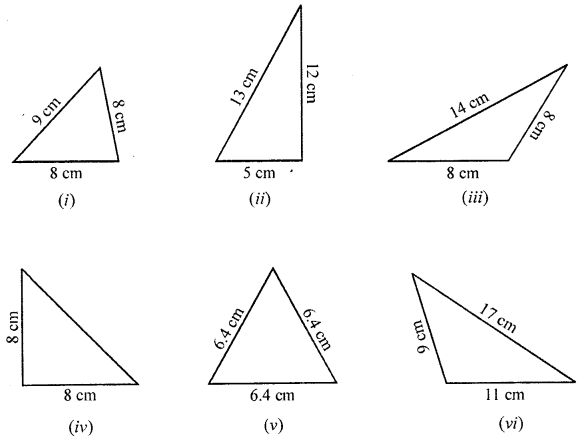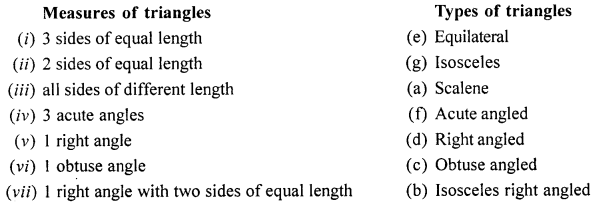## ML Aggarwal Class 6 Solutions for ICSE Maths Chapter 11 Understanding Symmetrical Shapes Ex 11.4

Question 1.
Name the following triangles with regards to sides :Solution:
(i) Isosceles triangle
(ii) Scalene triangle.
(iii) Equilateral triangle.

Question 2.
Name the following triangles with regards to angles :Solution:
(i) Right angled triangle as ∠B = 90°.
(ii) Obtuse angled triangle as ∠B is obtuse and greater then 90°.
(iii) Acute angled triangle as all the angle are less than 90°.

Question 3.
Name each of the following triangles in two different ways (you may judge the nature of the angle by observation):Solution:
(i) Acute angled and isosceles triangle
(ii) right angled and scalene triangle
(iii) Obtuse angled and isosceles triangle
(iv) Right angled and isosceles triangle
(v) Equilateral and acute angled triangle
(vi) Obtuse angled and scalene triangle

Question 4.
Match the following:Solution:Question 5.
State which of the following statement are true and which are false :
(i) A triangle can have two right angles.
(ii) A triangle cannot have more than one obtuse angle.
(iii) A triangle has atleast two actue angles.
(iv) If all the three sides of a triangle are equal, it is called a scalene triangle.
(v) A triangle has four sides.
(vi) An isosceles triangle is an equilateral triangle also.
(vii) An equilateral triangle is an isosceles triangle also.
(viii)An scalene triangle has all its angles equal.
Solution:
(i) False
(ii) True
(iii) True
(iv) False
(v) False
(vi) False
(vii) True
(viii) False